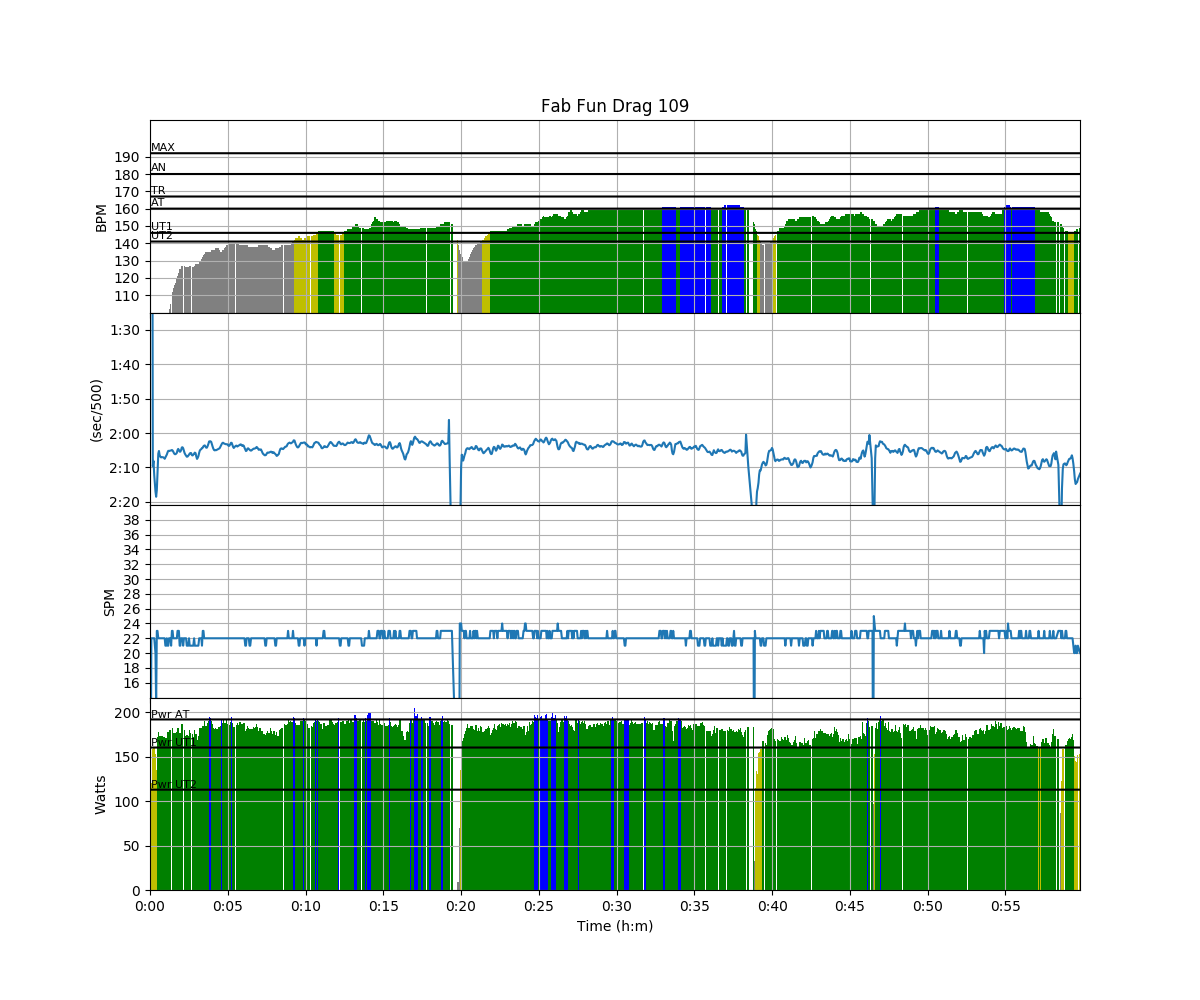## Rowing by numbers – big fun

To make it a bit more interesting, I added a mental arithmetic challenge.

After 1000m of rowing, I took the power of the stroke at which I crossed 1000m, in Watts, and subtracted 100. I multiplied that number by my heart rate minus 100.

I got

``````188-100 = 88
128-100 = 29
------------ x
3534
``````

So I rowed to 3534m, where I did the same arithmetic with the value at that moment:

``````3534 + (97x56) = 8964
``````

So I rowed to 8964m, where I had to calculate:

``````8964 + (86x62) = 14196
``````

In fact, I made errors on all the calculations, so the numbers above are just as an example (although the meter values are approximately right).

Anyway, it was good fun and I realized I need to restore my mental math skills. I am pretty good at estimating and I used to be slightly faster than gents with calculators, back when I was a Ph.D. student and estimating skills and mental arithmetic were a handy skill to have have.Yes, I do realize that this is not everybody’s idea of fun, but it works for me. The hour was over in no time.